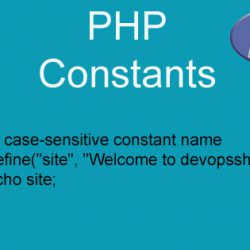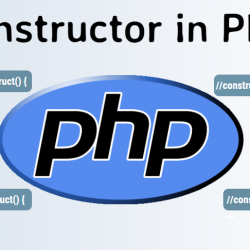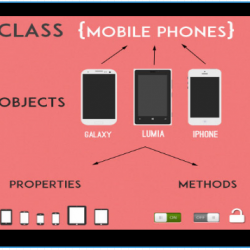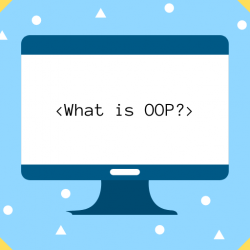## Complete Tutorials of PHP OOP Constants with Example code

In this Tutorial, we are going to learn about Complete Tutorials of PHP OOP Constants with Example code. Through this we will learn about the concept about Constants.  But first…## Complete Tutorials of PHP OOP Constructor with Example code

In this Tutorial, we are going to learn about Complete Tutorials of PHP OOP Constructor with Example code. Through this we will learn about the concept about constructor.  But first…## Complete Tutorials of PHP OOP Classes/Objects with Example code

The points of object-oriented programming in PHP are discussed in this article. We will begin with a class and object introduction and examine a few advanced concepts of such How,…